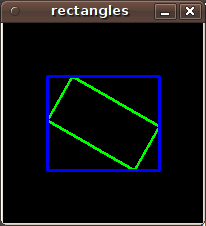OpenCV  3.2.0 Open Source Computer Vision
cv::RotatedRect Class Reference

The class represents rotated (i.e. not up-right) rectangles on a plane. More...

#include "types.hpp"

## Public Member Functions

RotatedRect ()
various constructors More...

RotatedRect (const Point2f &center, const Size2f &size, float angle)

RotatedRect (const Point2f &point1, const Point2f &point2, const Point2f &point3)

Rect boundingRect () const
returns the minimal up-right integer rectangle containing the rotated rectangle More...

Rect_< float > boundingRect2f () const
returns the minimal (exact) floating point rectangle containing the rotated rectangle, not intended for use with images More...

void points (Point2f pts[]) const

float angle

Point2f center

Size2f size

## Detailed Description

The class represents rotated (i.e. not up-right) rectangles on a plane.

Each rectangle is specified by the center point (mass center), length of each side (represented by cv::Size2f structure) and the rotation angle in degrees.

The sample below demonstrates how to use RotatedRect:

Mat image(200, 200, CV_8UC3, Scalar(0));
RotatedRect rRect = RotatedRect(Point2f(100,100), Size2f(100,50), 30);
Point2f vertices;
rRect.points(vertices);
for (int i = 0; i < 4; i++)
line(image, vertices[i], vertices[(i+1)%4], Scalar(0,255,0));
Rect brect = rRect.boundingRect();
rectangle(image, brect, Scalar(255,0,0));
imshow("rectangles", image);image
CamShift, fitEllipse, minAreaRect, CvBox2D
Examples:
fitellipse.cpp, and minarea.cpp.

## § RotatedRect() [1/3]

 cv::RotatedRect::RotatedRect ( )

various constructors

## § RotatedRect() [2/3]

 cv::RotatedRect::RotatedRect ( const Point2f & center, const Size2f & size, float angle )
Parameters
 center The rectangle mass center. size Width and height of the rectangle. angle The rotation angle in a clockwise direction. When the angle is 0, 90, 180, 270 etc., the rectangle becomes an up-right rectangle.

## § RotatedRect() [3/3]

 cv::RotatedRect::RotatedRect ( const Point2f & point1, const Point2f & point2, const Point2f & point3 )

Any 3 end points of the RotatedRect. They must be given in order (either clockwise or anticlockwise).

## § boundingRect()

 Rect cv::RotatedRect::boundingRect ( ) const

returns the minimal up-right integer rectangle containing the rotated rectangle

## § boundingRect2f()

 Rect_ cv::RotatedRect::boundingRect2f ( ) const

returns the minimal (exact) floating point rectangle containing the rotated rectangle, not intended for use with images

## § points()

 void cv::RotatedRect::points ( Point2f pts[] ) const

returns 4 vertices of the rectangle

Parameters
 pts The points array for storing rectangle vertices.
Examples:
fitellipse.cpp, and minarea.cpp.

## § angle

 float cv::RotatedRect::angle
Examples:
fitellipse.cpp.

## § center

 Point2f cv::RotatedRect::center
Examples:
fitellipse.cpp.

## § size

 Size2f cv::RotatedRect::size
Examples:
fitellipse.cpp.

The documentation for this class was generated from the following file: